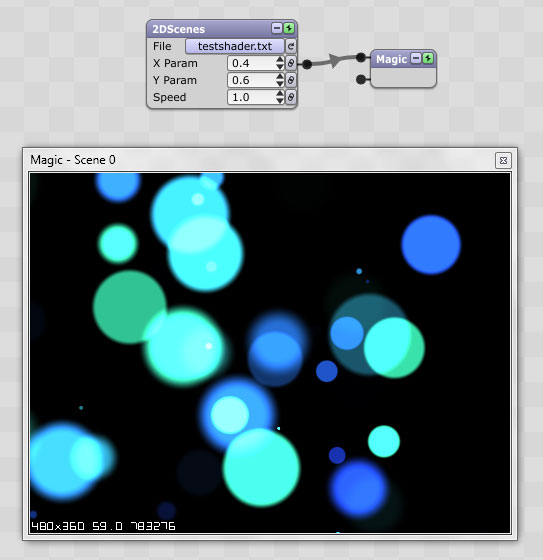Modifying GLSL shader files for the 2DScenes module

Modifying GLSL shader files for the 2DScenes module

EDIT: In Magic 1.5, the 2DScenes module has been superseded by the GLSLShader module. However, the tutorial below remains relevant.
---

When selecting different shader files for the 2DScenes module, you may notice that not all of the files connect to the X and Y parameters. In other words, changing the X and Y parameters has no effect on the graphics produced by some of the files.

In this post I'm going to try to demonstrate how to modify a file to make it connect to the X parameter so that the graphics can be dynamically linked to audio or MIDI. This is a bit of an "advanced" tutorial so bear with me.

I've gone to the GLSL Sandbox and chosen this file because I like how it looks and because I think it will be easy to modify:
http://glsl.heroku.com/e#18451.0

In case your browser doesn't support WebGL in the above link, this is what the shader looks like In Magic:To get the shader into Magic, I copied and pasted the entire text of the program into a file on my computer, which I called "testshader.txt".
Code: Select all
#ifdef GL_ES
precision mediump float;
#endif

// rakesh@picovico.com : www.picovico.com

uniform float time;
uniform vec2 mouse;
uniform vec2 resolution;

void main(void)
{
vec2 uv = -1.0 + 2.0*gl_FragCoord.xy / resolution.xy;
uv.x *=  resolution.x / resolution.y;

vec3 color = vec3(0.0);

// bubbles
for( int i=0; i<64; i++ )
{
// bubble seeds
float pha = tan(float(i)*6.+1.0)*0.5 + 0.5;
float siz = pow( cos(float(i)*2.4+5.0)*0.5 + 0.5, 4.0 );
float pox = cos(float(i)*3.55+4.1) * resolution.x / resolution.y;

// buble size, position and color
*mod(pha+0.1*(time/5.)*(0.2+0.8*siz),1.0)) * vec2(1.0, 1.0);
float dis = length( uv - pos );
vec3  col = mix( vec3(0.1, 0.2, 0.8), vec3(0.2,0.8,0.6), 0.5+0.5*sin(float(i)*sin(time*pox*0.03)+1.9));

// render
color += col.xyz *(1.- smoothstep( rad*(0.65+0.20*sin(pox*time)), rad, dis )) * (1.0 - cos(pox*time));
}

gl_FragColor = vec4(color,1.0);
}

You'll notice that, in the file, there is a line that says:
Code: Select all

If you change that value, let's say to 0.05, and then you reload the shader file, you'll notice that the circles get smaller:
Code: Select allWhat I'd like to do is make it so that I can control the radius of the circles dynamically with audio or MIDI in Magic.

For any shader program, Magic will automatically connect to the variable in the program called "mouse":
Code: Select all
uniform vec2 mouse;

Thus, the task at hand is to get rid of this line:
Code: Select all

And replace any references to it with the mouse variable. Specifically in this case, I want to replace it with "mouse.x", since this will connect to the X parameter of the 2DScenes module.

Fortunately, in this program it's pretty easy. The only reference to fRadius is here:
Code: Select all

So all I have to do is make this change:
Code: Select all
float rad = mouse.x + sin(float(i))*0.12+0.08;

I'm also going to make one minor change because I don't want the radius to be influenced by anything other than the mouse value. So I'll delete everything else on that line:
Code: Select all

And that's it! Reload the file in Magic, and now you can use the X param to set the radius:Here I've linked the X param to Volume. You can see that the radius is small because the Volume is very low at this moment:So, I hope that helps everyone out a bit. Here's the final modified code for the file. Notice that I've deleted the fRadius line entirely because it isn't needed.
Code: Select all
#ifdef GL_ES
precision mediump float;
#endif

// rakesh@picovico.com : www.picovico.com

uniform float time;
uniform vec2 mouse;
uniform vec2 resolution;

void main(void)
{
vec2 uv = -1.0 + 2.0*gl_FragCoord.xy / resolution.xy;
uv.x *=  resolution.x / resolution.y;

vec3 color = vec3(0.0);

// bubbles
for( int i=0; i<64; i++ )
{
// bubble seeds
float pha = tan(float(i)*6.+1.0)*0.5 + 0.5;
float siz = pow( cos(float(i)*2.4+5.0)*0.5 + 0.5, 4.0 );
float pox = cos(float(i)*3.55+4.1) * resolution.x / resolution.y;

// buble size, position and color
*mod(pha+0.1*(time/5.)*(0.2+0.8*siz),1.0)) * vec2(1.0, 1.0);
float dis = length( uv - pos );
vec3  col = mix( vec3(0.1, 0.2, 0.8), vec3(0.2,0.8,0.6), 0.5+0.5*sin(float(i)*sin

(time*pox*0.03)+1.9));

// render
color += col.xyz *(1.- smoothstep( rad*(0.65+0.20*sin(pox*time)), rad, dis )) * (1.0 -

cos(pox*time));
}

gl_FragColor = vec4(color,1.0);
}
Eric